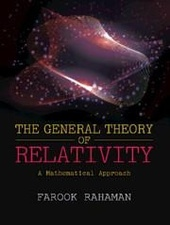# THE GENERAL THEORY OF RELATIVITY

A Mathematical Approach

Cambridge University Press
• erscheint ca. am 28. Februar 2021

• Buch
• |
• Hardcover
• |
• 400 Seiten
978-1-108-83799-6 (ISBN)

The book aims to expound the general theory of relativity with a mathematical point of view. Catering to the needs of postgraduate students and researchers in the field of astrophysics and mathematical physics, it offers the readers a comprehensive understanding of the advanced topics of the subject matter. It specifically discusses the mathematical foundation of tensor calculus, gives a background of geodesics, Einstein's field equations, linearised gravity, spacetime of spherically symmetric distribution of matter and black holes, and particle and photon orbits in spacetime. Apart from the formulation of general relativity, Lie derivatives and its applications, and causality of spacetime are also discussed in detail. Certain preliminary concepts of extrinsic curvature, Lagrangian formalism of general theory of relativity and 3 + 1 decomposition of space-time are covered and are provided in the book as appendices.
 Sprache: Englisch Verlagsort: Cambridge | Großbritannien Zielgruppe: Für Beruf und Forschung Illustrationen: Worked examples or Exercises Schlagworte: ISBN-13: 978-1-108-83799-6 (9781108837996)weitere Ausgaben werden ermittelt
Dr Farookh Rahaman is Professor of Mathematics at Jadavpur University, Kolkata. He has published numerous research papers on galactic dark matter, wormhole geometry, charged fluid model, topological defects in the early universe, gravastars, black hole physics, star modelling, and the cosmological model of the Universe. He has co-authored a book entitled The Special Theory of Relativity (2014).
Preface; Acknowledgement; 1. Tensor calculus - a brief overview; 2. Geodesics; 3. Einstein field equations; 4. Linearized gravity; 5. Lie derivatives and Killing's equation; 6. Spacetimes of spherically symmetric distribution of matter and black holes; 7. Particle and photon orbits in the Schwarzschild spacetime; 8. Causal structure of spacetime; 9. Exact solutions of Einstein equations and their causal structures; 10. Rotating Black holes; 11. Elementary cosmology; 12. Elementary astrophysics; Appendix A. Extrinsic curvature; Appendix B. Lagrangian formalism of general relativity; Appendix C. 3+1 decomposition; Bibliography; Index.
Schweitzer Klassifikation
DNB DDC Sachgruppen
Dewey Decimal Classfication (DDC)
• Science
•Physics
•Physics
BIC Classifikation
• Mathematics & science
•Physics
•Applied physics
•Astrophysics
• Mathematics & science
•Physics
•Mathematical physics
• Mathematics & science
•Physics
•Relativity physics
BISAC Classifikation
• Science
•Astronomy
•Cosmology

Noch nicht erschienen

 61,84 € inkl. 5% MwSt.